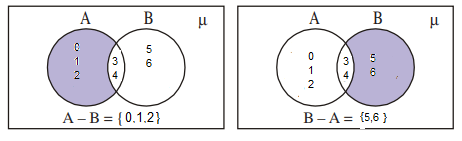# AP SSC Class 10 Maths Chapter 2 Sets

The AP SSC Class 10 Maths Chapter 2 Sets discusses the different types of set and the representation of a set using a Venn diagram. In this article, let us understand what a set is and solve a few problems related to sets.

## What is a Set?

A well-defined collection of objects is known as a set. By well defined, we mean

• Presence of a common feature or property in all the objects of a set
• A clear distinction of whether a given object belongs to the set or not.

A set is written in a roster form where all elements of a set are written, separated by commas, within { } curly brackets. A set that does not contain any element is known as a Null set. Venn diagrams are used to represent various functions between sets.

## AP Class 10 Maths Chapter 2 Sets Questions

1. Let A = {0, 1, 2, 3, 4}; B = {3, 4, 5, 6}. Find A – B. Show the Venn diagram representation.

Solution:

For A – B, consider the elements which are in A but not in B.

Therefore, A – B ={0,1,2}

Similarly, for B – A consider the elements which are in B but not in A.

Therefore, B – A = {5,6}

Hence, we can conclude that A – B ≠ B – A

The Venn diagram representation of A – B and B – A is given as1. If A and B are two sets such that A ⊂ B then what is A ∪ B?

Solution:

Let us consider A ={1,2} and B={1,2,3},

Every element of A is in B, hence A ⊂ B.

A ∪ B = {1,2} ∪ {1,2,3}

= {1,2,3}

A ∪ B = B

3. Write the following in roster and set builder forms.
(i) The set of all natural numbers which divide 42.
(ii) The set of natural numbers which are less than 10.

Solutions: (i) Given that B be the set of all natural numbers that divide 42.
B = {1, 2, 3, 6, 7, 14, 21, 42} Roster form and
B = {x : x is a natural number that divides 42} Set builder form

Then,
(ii) If A is the set of all natural numbers less than 10, we can write:
A = {1, 2, 3, 4, 5, 6, 7, 8, 9} (Roster form) and
B = {x : x is a natural number which is less than 10} (Set builder form)

Stay tuned to BYJU’S to get the latest notification on SSC exam along with AP SSC model papers, exam pattern, marking scheme and more.